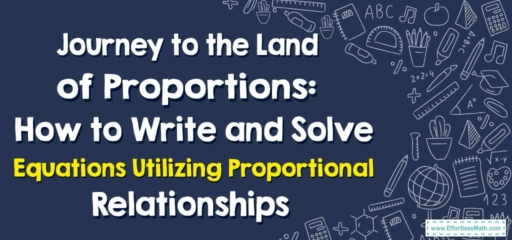# Journey to the Land of Proportions: How to Write and Solve Equations Utilizing Proportional Relationships

We're embarking on a new adventure, dear reader, a journey into the world of ratios and proportions! Sit tight as we navigate the interesting terrain of proportional relationships, applying our skills to write and solve equations.## 1. Understanding Proportional Relationships

Proportional relationships are a vital part of our mathematical toolkit. In a nutshell, they describe a relationship where the ratio of two variables is constant. If you double one, the other doubles too. If you halve one, the other halves as well.

## 2. Traversing the Terrain: Writing Equations with Proportional Relationships

### A Map to Success: Writing Equations Utilizing Proportional Relationships

#### Step 1: Understand the Problem

Our adventure begins by comprehending the situation. What are we dealing with? Are we trying to find out how far a car can travel at a constant speed? Or how many batches of cookies we can bake with a certain amount of ingredients?

#### Step 2: Identify the Proportion

We have our situation. Now, we look for the proportional relationship, usually presented as a ratio or a rate.

#### Step 3: Write the Equation

Using our proportion, we can write our equation. If $$y$$ is proportional to $$x$$, we write the equation as $$y = kx$$, where k is the constant of proportionality or the value that remains the same in our proportional relationship.

## 3. The Climax of our Adventure: Solving Proportional Equations

### Conquering the Peaks: Solving Equations Utilizing Proportional Relationships

#### Step 1: Set Up the Equation

First, we place our equation from the previous steps on the battlefield.

#### Step 2: Substitute Given Values

Our weapons in this fight are the values we know. We substitute them into the equation.

#### Step 3: Solve for the Unknown

Armed with our values and equation, we can solve for the missing piece, finding our unknown.

Let’s illustrate this with an example: If a car travels $$100$$ miles in $$2$$ hours at a constant speed, how far will it travel in $$5$$ hours?

1. Understand the Problem: We’re discovering how far a car can travel in 5 hours at a constant speed.
2. Identify the Proportion: The car travels $$100$$ miles in $$2$$ hours.
3. Write the Equation: The proportion can be written as $$y = 50x$$, where y is the distance and $$x$$ is the time.
4. Set Up the Equation: Now, we set up our equation: $$y = 50\times 5$$.
5. Substitute Given Values: We substitute $$5$$ for $$x$$, our time.
6. Solve for the Unknown: Solving our equation, we find that $$y = 250$$. The car will travel $$250$$ miles in $$5$$ hours.

There you have it, adventurers! You’ve journeyed through the landscape of proportional relationships, mastering the art of writing and solving proportional equations. Use this power wisely, and keep exploring the vast expanse of mathematics!

### What people say about "Journey to the Land of Proportions: How to Write and Solve Equations Utilizing Proportional Relationships - Effortless Math: We Help Students Learn to LOVE Mathematics"?

No one replied yet.

X
30% OFF

Limited time only!

Save Over 30%

SAVE $5 It was$16.99 now it is \$11.99